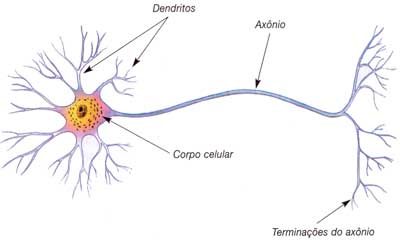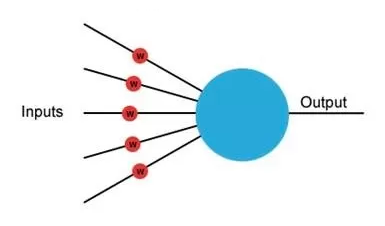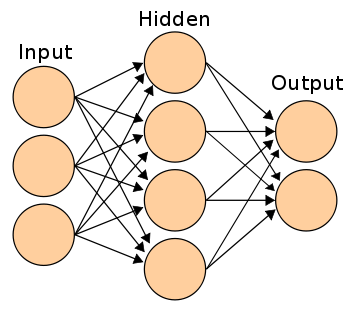# Isabel Gomes

Studies computer engineering at University of São Paulo and loves athletics, especially shot put. Aims to become a professional programmer.

•Overview of Neural Networks
•Machine Learning course on Coursera

Overview of Neural Networks

Overview

The idea of neural network algorithm came as an attempt to mimic the brain and its amazing ability to learn. Although it is a relatively old idea (emerged around the years 80-90), nowadays neural networks is considered the state of the art in many applications.

Neural network algorithms are based on the hypothesis that the brain has only one algorithm that can learn all features of the body, i.e., any area of the brain could learn to see or hear if it received the appropriate stimulus.

Representation

In the brain, each neuron receives nerve impulses through dendrites, performs some "calculation" in cell body and transmits the response via another nerve impulse using the axon. A neural network algorithm copies this system, as shown in Figures 1 and 2 below.##### Figures 1 and 2 - representation of a neuron (left) and a neural network unit (right).

In this type of algorithm, several "neurons" are interconnected to form a network. This network consists of three types of layers, known as input layer, output layer and hidden layers, as shown in Figure 3 below.##### Figure 3 - representation of a neural network.

The input layer receives the data and the output layers outputs the response. The hidden layers are responsible for some intermediate calculation that helps the network to find the final answer. In more complex networks, one can use multiple hidden layers between the input and the output layers. The number of neurons in each layer depends on the amount of input data and the type of problem being solved. For example, if the algorithm was designed to determine whether or not a patient has a specific disease, the input layer has as many neurons as the number of features in the input data and the output layer has only one neuron.

Machine Learning course on Coursera

Recently, I've decided to take an online class about Machine Learning at Coursera. In this article, I will talk about the structure of the course and its content.

#### Class goal

The Machine Learning class is taught by Andrew Ng, co-founder of Coursera and director of the Artificial Intelligence laboratory of Stanford University.

The class goal is teaching some algorithms that solve problems using artificial intelligence, as well as to present the intuition behind them.

#### Methodology

The class is divided in 10 lessons about different machine learning algorithms. Each class consists of:

• Videos: explain the operation and the application of the algorithm and also give usage examples;
• Review questions: test the student's understanding about the subjects explained in the videos;
• Programming exercises: apply in a straightforward manner the concepts studied in the class and help to better understand them.

#### Algorithms

The algorithms taught in the class can be divided into the following categories:

1. Supervised learning
2. Unsupervised learning

Supervised learning refers to algorithms that try to predict a result based on a data set. In these cases, in order to train the algorithm, it is necessary to feed it with a database that has the inputs and the corresponding outputs. This type of machine learning algorithms are divided into two subcategories:

• Regression problems: in this case, the algorithm's output values are continuous, i.e., they can take an infinite number of values. For example, the decision of a house price based on its characteristics, such as the number of bedrooms and its size, is considered to be a regression problem.
• Classification problems: the output of algorithms of this subcategory are discrete values contained in a defined set of values. For example, an algorithm that decides whether an email is spam or not always outputs 0 or 1, so it can be considered a classification algorithm.

Unsupervised learning refers to algorithms that try to group together data that have similar characteristics. For example, this type of algorithm can be used to group together similar people in social networks.

In this course, the main supervised learning algorithms taught are Linear Regression, Logistic Regression, Neural Networks and Support Vector Machine, and the main unsupervised learning algorithms are K-Means, Anomaly detection and Recommender Systems.